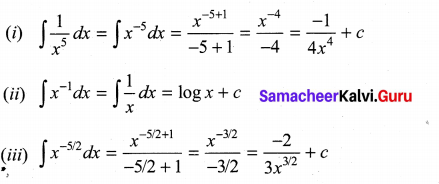## Tamilnadu Samacheer Kalvi 11th Maths Solutions Chapter 11 Integral Calculus Ex 11.1

Integrate the following with respect to ‘x’:
Question 1.
(i) x11
(ii) $$\frac{1}{x^{7}}$$
(iii) $$\sqrt{x^{4}}$$
(iv) $$\left(x^{5}\right)^{\frac{1}{8}}$$
Solution: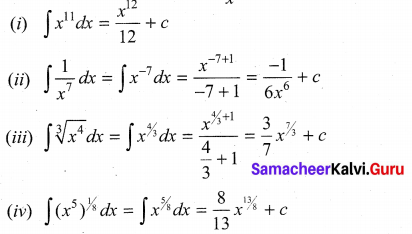Question 2.
(i) $$\frac{1}{\sin ^{2} x}$$
(ii) $$\frac{\tan x}{\cos x}$$
(iii) $$\frac{\cos x}{\sin ^{2} x}$$
(iv) $$\frac{1}{\cos ^{2} x}$$
Solution: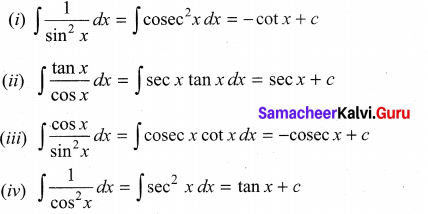Question 3.
(i) 123
(ii) $$\frac{x^{24}}{x^{25}}$$
(iii) ex
Solution: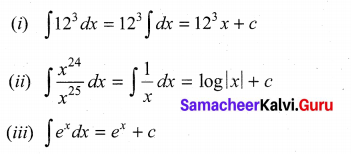Question 4.
(i) (1 + x2)-1
(ii) $$\left(1-x^{2}\right)^{-\frac{1}{2}}$$
Solution: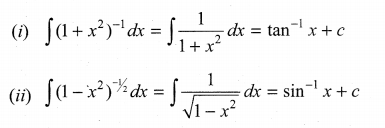### Samacheer Kalvi 11th Maths Solutions Chapter 11 Integral Calculus Ex 11.1 Additional Problems

Integrate the following with respect to x.
Question 1.
(i) $$\sqrt{x^{7}}$$
(ii) (x10)1/7
Solution: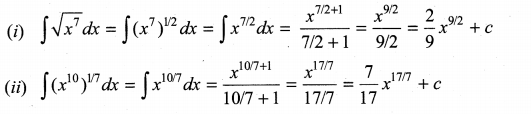Question 2.
(i) $$\frac{1}{x^{5}}$$
(ii) x-1
(iii) $$\frac{1}{x^{5 / 2}}$$
Solution: